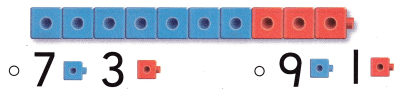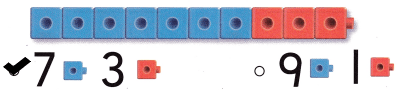# Texas Go Math Kindergarten Module 5 Assessment Answer Key

Refer to our Texas Go Math Kindergarten Answer Key Pdf to score good marks in the exams. Test yourself by practicing the problems from Texas Go Math Kindergarten Module 5 Assessment Answer Key.

## Texas Go Math Kindergarten Module 5 Assessment Answer Key

Concepts and Skills

DIRECTIONS: 1-2. Count and tell how many yellow counters. Write the number. Count and tell how many red counters. Write the number. (TEKS K.2.B)

Question 1.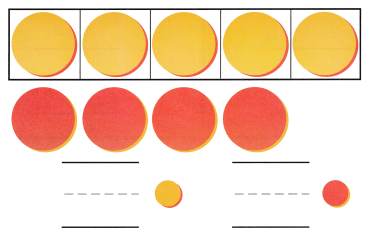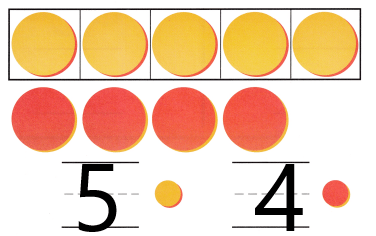Explaination:
There are 5 yellow counters and 4 red counters. so, i wrote the numbers 5 and 4.

Question 2.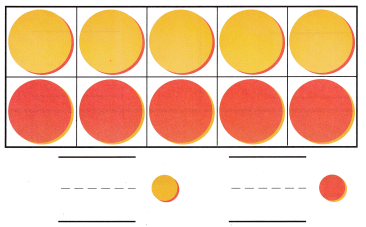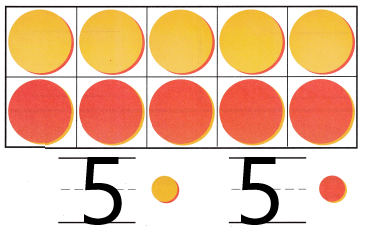Explaination:
There are 5 yellow counters and 5 red counters. so, i wrote the numbers 5 and 5.

DIRECTIONS: 3-6. Count the objects. Write the number. (TEKS K.2.B) 7. Choose the correct answer. Which shows how many blue and red cubes? (TEKS K.2.I)

Question 3.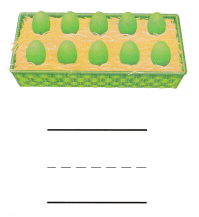Explaination:
I counted and wrote number 10 as there are 10 eggs.

Question 4.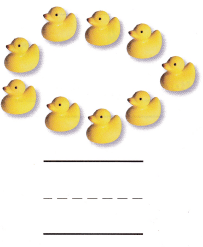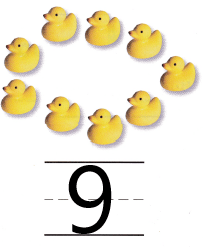Explaination:
I counted and wrote number 9 as there are 9 ducks.

Question 5.Explaination:
I counted and wrote number 9 as there are 9 counters.

Question 6.Explaination:
I counted and wrote number 10 as there are 10 counters.

Question 7.
Texas Test Prep